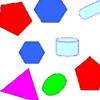#### You may also like### Bracelets

Investigate the different shaped bracelets you could make from 18 different spherical beads. How do they compare if you use 24 beads?### Cut and Make

Cut a square of paper into three pieces as shown. Now,can you use the 3 pieces to make a large triangle, a parallelogram and the square again?### Part the Polygons

Draw three straight lines to separate these shapes into four groups - each group must contain one of each shape.

# Is a Square a Rectangle?

### Why do this problem?

The lead question for this problem, "How many rectangles can you find in this shape?" is an ideal way to introduce children to consider the properties of rectangles. The aim is not to simply count the number of rectangles but to discuss what a rectangle is.

The problem also looks at whether the rectangles are similar.

For more discussion on the properties of rectangles, see this sheet.

### Key questions

Can you tell me what a rectangle is?
Did you know that a square is a special sort of rectangle? What is the difference between a square and a rectangle?
How many rectangles did you find in the shape? Which ones are the same and which ones are different?
How many squares did you find?

### Possible extension

Learners who found this very easy could try this problem.

### Possible support

Suggest using coloured pencils to help in counting the different rectangles.